# Validity area of several simultaneos linear inequations

In this section we will explain how to solve the following type of problems:

Given several restrictions (inequations), we have to determine the area on the plane that satisfies all of them by giving its vertexes.

We usually find more than one simultaneous restriction for the variables in inequations exercises. For example, if we have to find the number of chairs (of $$10$$ kg each) and tables (of $$20$$ kg each) that can be carried by a truck which cannot carry more than $$1000$$ kg, we have to consider the restriction that the number of chairs and tables has to be positive. Therefore, we do not only have to consider the weight restriction for the truck:

(i) $$10\cdot x+20\cdot y\leqslant 1000$$

but also restrictions of being positive both the number of chairs ($$x$$) and the number of tables ($$y$$):

(ii) $$x\geqslant0$$

(iii) $$y\geqslant0$$

Each of these restrictions has a straight line associated with the plane XY, that separates the plane in two regions: the validity region (region where the restriction is satisfied) and the area in where it is not satisfied. Next for these straight lines and areas of validity, there are three restrictions:

(i) The restriction is: $$10\cdot x+20\cdot y\leqslant 1000$$$and therefore the associated straight line is: $$f(x)=-\dfrac{1}{2}\cdot x+50$$$

If we also try the point $$(x=0,y=0)$$ in the inequation:

$$10\cdot 0+20\cdot 0\leqslant 1000$$$therefore the validity region will be the one that contains the point $$(0,0)$$: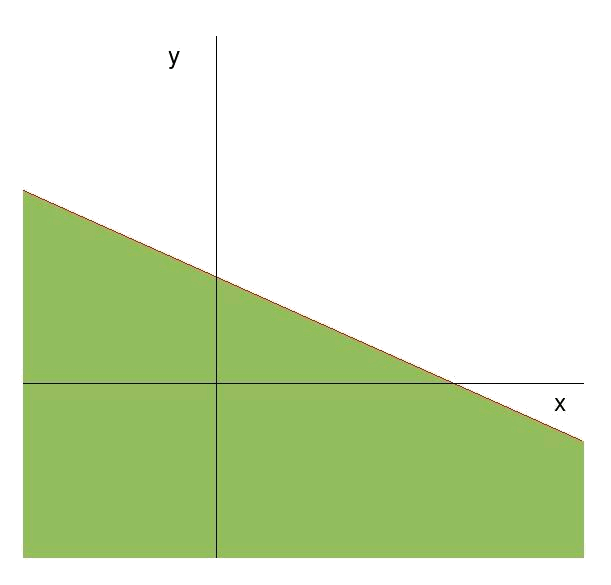(ii) The restriction is: $$x\geqslant0$$$

This type of restriction represent a vertical straight line (parallel to the axis $$y$$) that separates the values of $$x$$ greater than and less than $$0$$ respectively. The values will be the validity area of $$x$$ greater than zero: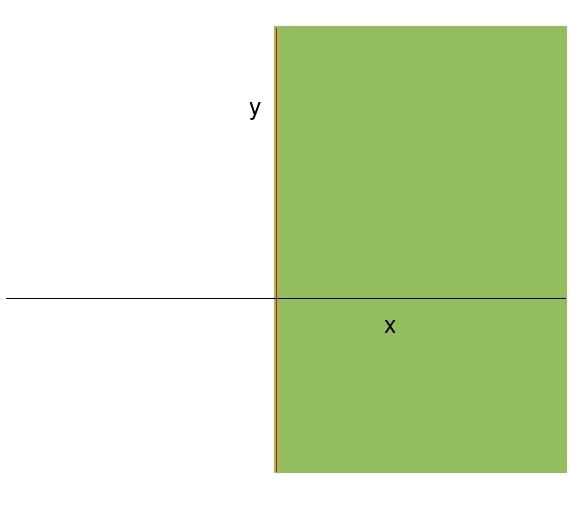iii) The restriction is: $$y\geqslant0$$$The straight line associated with this restriction is: $$g(x)=0$$$

and the validity area is, obviously, the region over $$g(x)$$, $$\ y\geqslant0$$: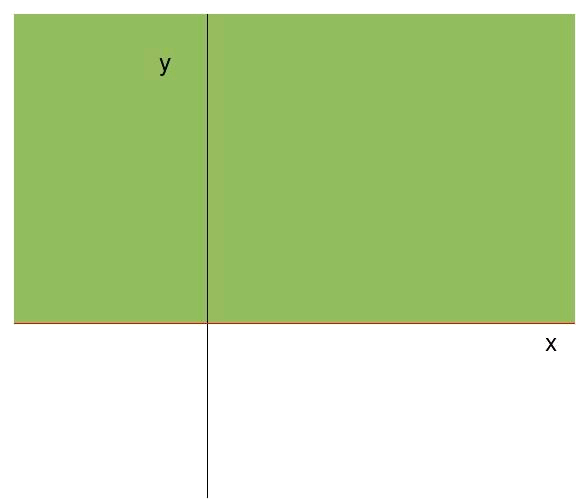Now we should know the region of the plane XY where all the restrictions are satisfied simultaneously. This region will the one that is common to the validity regions that are free of restrictions. For the case of the chairs and the tables it will be the triangle composed by the straight line $$f(x)$$ and the axes $$x$$ and $$y$$: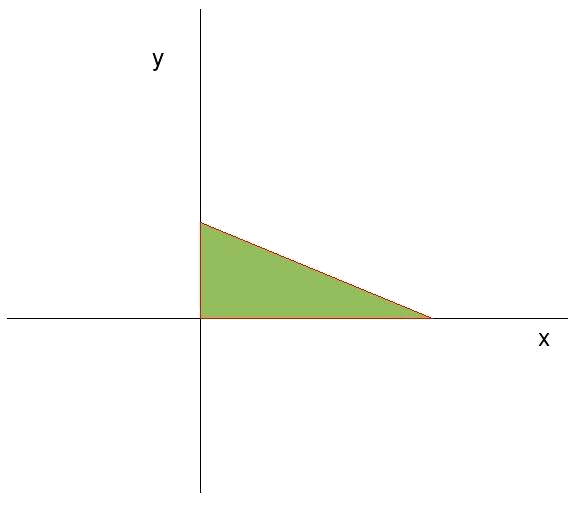We can see that in this case, as we take into account all the restrictions simultaneously, the validity region (from now on we will refer to the common region of all the areas of validity from the different restrictions simply as the validity region) is an enclosed area of the plane. In the previous examples the validity region was spreading over some side up to infinity, that's why these areas were not bounded.

To know the validity region well the coordinates of its apexes have to be known. In this case it is very simple. We already know the coordinates of one of the points: $$(0,0)$$. The other two intersection points are the points where the straight line $$f(x)$$ cross with the axes.

The intersection points with the axes can be found easily:

• For the cross point with the axis $$y$$, we only have to know that the whole axis $$y$$ has a coordinate $$x=0$$, and the value of $$y$$ in the intersection point will be the one that takes the function $$f(x)=-\dfrac{1}{2}\cdot x+50$$ in $$x=0$$ (on the axis). So the intersection point will be: $$(x=0,y=f(0)=50)$$.

• And the intersection point with the axis $$x$$ is when $$y = 0$$, that is to say, in the value of $$x$$ where the function takes the value $$0$$: $$f(x)=0 \Rightarrow -\dfrac{1}{2}\cdot x+50=0 \Rightarrow x=100$$$and therefore the point where the straight line $$f(x)$$ crosses the axis $$x$$ is $$(x=100,y=0)$$. So the apexes of the region of validity have as their coordinates: $$(0,0) \quad (0,50) \quad (100,0)$$$

In this case it has been very easy to find the apexes.

The following example will illustrate the most general way to find the apexes of the region of validity.

We have the following restrictions: $$\begin{array}{rcl} x+4 &\geqslant& 4 \\ y &\leqslant& 4 \\ y &\geqslant& x \end{array}$$$that they take as associated straight lines: $$\begin{array}{l} r(x): \ y=-x+4 \\ s(x):\ y=4 \\ t(x):\ y=x \end{array}$$$

We can visualize these straight lines and determine the semiplanes where every inequation is satisfied separately.

For the straight line $$r$$: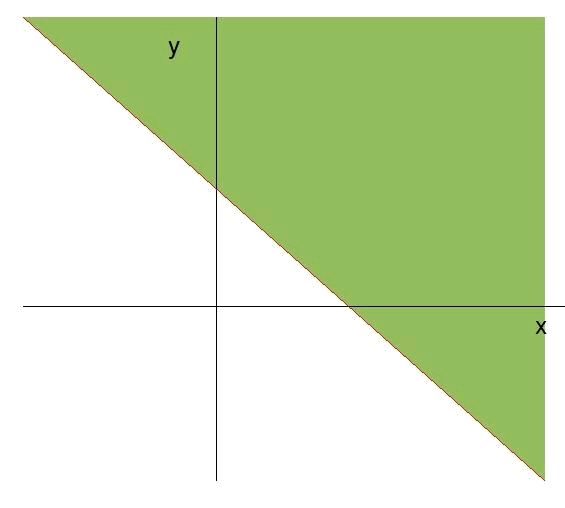Since the inequation is not satisfied at point $$(0,0)$$, $$\ 0+0\ngeqslant 4$$, we see that the area of validity of the inequation is the semiplane over the straight line.

For the straight line $$s$$: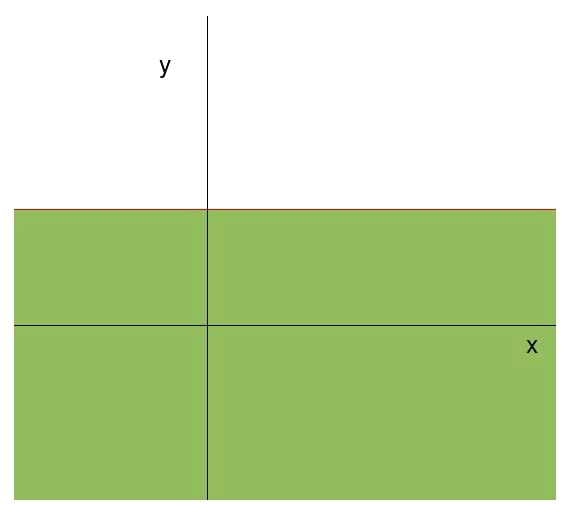Since the inequation is satisifed at point $$(0,0)$$, $$\ 0\leqslant 4$$, we see that the area of validity of the inequation is the semiplane below the straight line.

For the straight line $$t$$: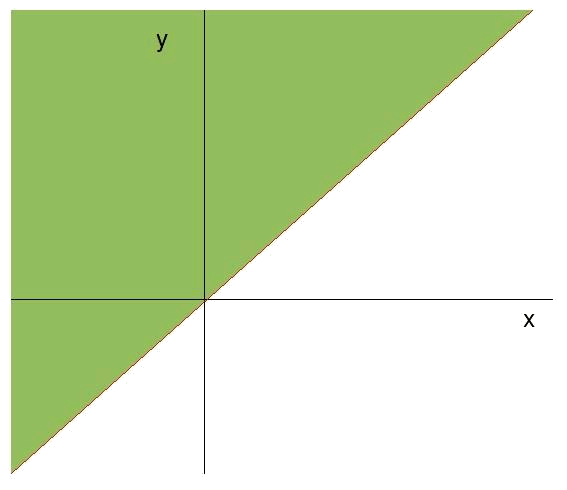Since the inequation is satisifed at point $$(0,1)$$, $$\ 1\geqslant 0$$ we see that the area of validity of the inequation is the semiplane over the straight line.

As a whole we have: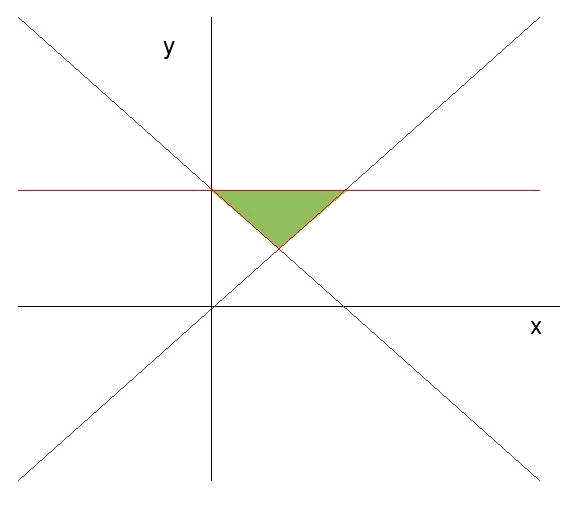The area where all the semiplanes coincide is the feasible region. We see that in this case it is also a question of a bounded area.

Now the calculation of the apexes of this area has to be done. To do so, it will be necessary to know the point where the straight lines cross. We will have to find three intersection points: the one of the straight line $$r$$ with $$s$$, the one of $$r$$ with $$t$$ and the one of $$s$$ with $$t$$.

How to find the point of intersection of two straight lines:

To know a point means to know the coordinates $$x$$ and $$y$$ of the above mentioned point. If two straight lines $$f(x)$$ and $$g(x)$$ cross, we know that both functions will be taking the same value in the position where they cross. Graphically it is: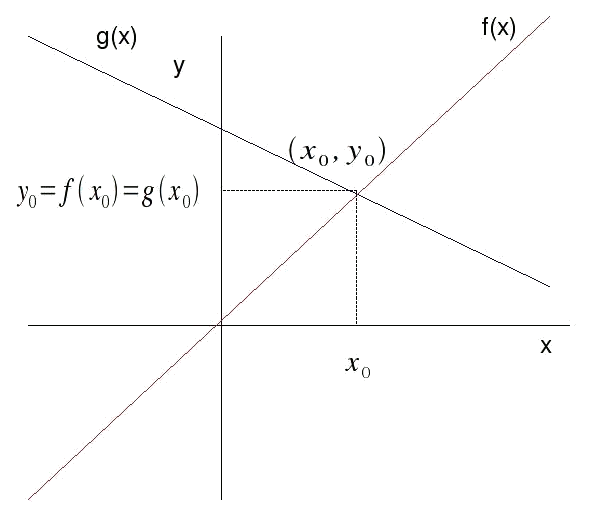Therefore we know that the intersection point will be $$(x_0,y_0)$$ and that the value of $$y_0$$ equals the value of two functions in $$x_0$$, since we know that two functions have to take the same value at this point in order to cross: $$f(x_0)=g(x_0)$$$Back to the example. Intersection point between $$r$$ and $$s$$: We have to find the coordinates $$x$$ and $$y$$ of the intersection point. We will call the coordinates of this point $$x_0$$ and $$y_0$$. To find the coordinate $$x$$ where the straight lines cross, $$x_0$$, we equal two functions $$r(x)=-x+4$$ and $$s(x)=4$$ at the point where the straight lines cross ($$x_0$$): $$r(x_0)=s(x_0) \Rightarrow -x_0+4=4 \Rightarrow x_0=0$$$

Therefore two straight lines cross at $$x_0=0$$.

Determining the coordinate $$y$$ of the intersection point $$y_0$$ is simple, for it is the value that both functions $$r(x)$$ and $$s(x)$$ take in $$x=x_0$$.

$$y_0=r(x_0)=s(x_0) \Rightarrow y_0=r(0)=s(0)=-0+4=4$$$Therefore the intersection point between the straight lines $$r$$ and $$s$$ is: $$(x_0=0,y_0=4)$$. Intersection point between $$r$$ and $$t$$: We proceed just as in the previous case. We equal the functions $$r(x)=-x+4$$ and $$t(x)=x$$ at the point of intersection, that this time will take as its coordinates $$(x_1,y_1)$$. $$r(x_1)=t(x_1) \Rightarrow -x_1+4=x_1 \Rightarrow x_1=2$$$

As in the previous case, the coordinate $$y$$ of the intersection point, $$y_1$$, is equal to the value that the functions $$r(x)$$ and $$t(x)$$ take at the intersection point:

$$y_1=r(x_1)=t(x_1)=-2+4=2$$$And so, the coordinates of the point of intersection are: $$(x_1=2,y_1=2)$$. Intersection point between $$s$$ and $$t$$: This intersection point will have as its coordinates $$(x_2,y_2)$$. First we determine the value of $$x_2$$ as in the previous cases, that is to say by equaling $$s(x)=4$$ and $$t(x)=x$$ at the intersection point $$x_2$$: $$s(x_2)=t(x_2) \Rightarrow 4=x_2$$$

We decide the value of the coordinate $$y$$ of the point of intersection, $$y_2$$, as in the previous occasions: $$y_2=s(x_2)=t(x_2)=4$$$Therefore the intersection point between the straight lines $$s(x)$$ and $$t(x)$$ is the one that takes as its coordinates: $$(x_2=4,y_2=4)$$. In short, the coordinates of the apexes of the feasible region are: $$(x_0,y_0)=(0,4) \quad (x_1,y_1)=(2,2) \quad (x_2,y_2)=(4,4)$$$

Other examples:

Considering the following inequations system: $$\begin{array}{rcl} x &\geqslant& 0 \\ y &\leqslant& 4 \\ y &\geqslant& \dfrac{x}{2} \end{array}$$$We look first for the straight lines associated with every inequation and the areas of validity of each one: • The first of them gives us a straight line parallel to the axis $$y$$ in $$x=0$$ and its validity region is the one that has $$x$$ greater than $$0$$ (towards the right of the axis $$y$$). • The second one is a straight line parallel to the axis $$x$$, that meets the point $$y=4$$ and takes as a validity region the semiplane that it has below (where $$y$$ is less than $$4$$). • The third straight line is $$y=\dfrac{x}{2}$$ and its validity region is the one that is above the straight line (it is possible to verify this easily, seeing that the point $$(0,1)$$ satisfies the inequation: $$1\geqslant$$).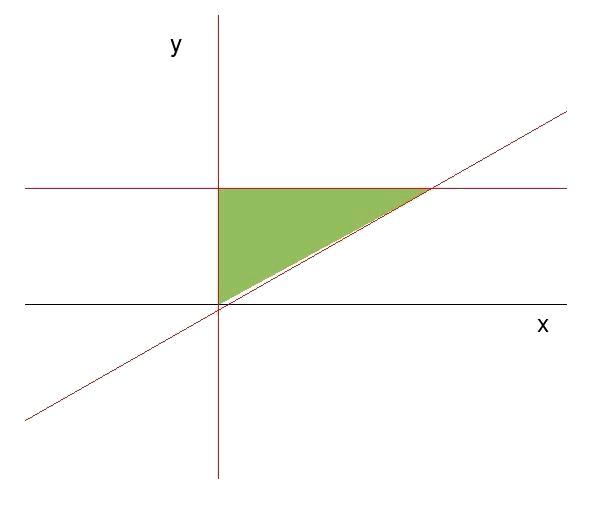We will determine the apexes of the area of validity as the intersection points between the different straight lines. • The straight line $$x=0$$ crosses with $$y=4$$ at point $$(x_0=0,y_0=4)$$. • The straight line $$x=0$$ crosses with $$y=\dfrac{x}{2}$$ at point $$(x_1=0,y_1=0)$$. • The straight line $$y=4$$ crosses with $$y=\dfrac{x}{2}$$ at point $$(x_2=8,y_2=0)$$. Therefore, the apexes of the region of validity are: $$(x_0,y_0)=(0,4) \quad (x_1,y_1)=(0,0) \quad (x_2,y_2)=(8,0)$$$

Given the set of restrictions:

$$\begin{array}{rcl} x+3 &\geqslant& y \\ 8 &\geqslant& x+y \\ y &\geqslant& x-3 \\ x &\geqslant& 0 \\ y &\geqslant& 0 \end{array}$$$We look first for the straight lines associated with every restriction and the areas of validity of every inequation (checking it with a point in the inequations). The associatd straight lines are: $$\begin{array}{l} f: \ y=3+x \\ g:\ y=-x+8 \\ h:\ y=x-3 \\ i:\ x=0 \\ j:\ y=0 \end{array}$$$

Drawing the straight lines and the validity areas we can visualize the validity region.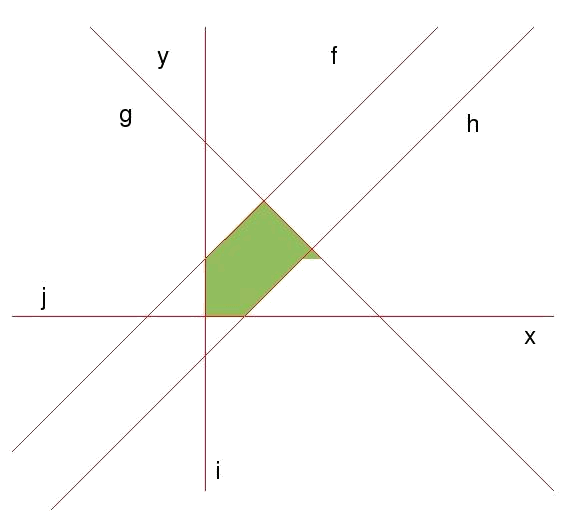If we cannot do the drawing we have an alternative. Having so many straight lines, normally we will have more intersection points between the straight lines than apexes in the validity area. For this reason, not all the points of intersection between the different straight lines will be apexes of the region of validity. To admit which ones are the apexes of the region of validity the following will be done:

• All the intersection points are calculated between the different straight lines.

• Those points of intersection where all the restrictions are fulfilled simultaneously will be the apexes of the area of validity (what can allow us to visualize it, if we have not done so before).

Thus, we are going to calculate all the intersection points between the different straight lines.

• $$f$$ with $$g$$ will cross at point $$(x_0,y_0)$$. We calculate the coordinates of the point as has already been done before: $$f(x_0)=g(x_0) \Rightarrow 3+x_0=-x_0+8 \Rightarrow x_0=\dfrac{5}{2}$$$And the coordinate $$y$$: $$y_0=f(x_0)=g(x_0)=\dfrac{11}{2}$$$ Therefore the coordinates of the point of intersection are: $$(x_0,y_0)=(\dfrac{5}{2}, \dfrac{11}{2})$$.

• $$f$$ with $$h$$ will cross at point $$(x_1,y_1)$$. We calculate the coordinates of the point as has already been done before: $$f(x_1)=h(x_1) \Rightarrow 3+x_1=x_1-3 \Rightarrow 3=-3$$$We see that, when we try to find the coordinate x at the intersection point, an equation that is not satisfied appears. This means that two straight lines are in fact parallel (therefore they never cross). • $$f$$ with $$i$$ will cross at point $$(x_2,y_2)$$. The straight line $$f(x)=3+x$$ will cross the straight line $$x=0$$ (straight line that coincides with the axis $$y$$) with point $$(x=0,y=f(0))$$. That is to say: $$(x_2,y_2)=(0,3)$$. • $$f$$ with $$j$$ will cross at point $$(x_3,y_3)$$. We calculate the coordinates of the point as has already been done before: $$f(x_3)=j(x_3) \Rightarrow 3+x_3=0 \Rightarrow x_3=-3$$$ And the coordinate $$y$$, $$y_3=f(x_3)=j(x_3)=0$$$Therefore the coordinates of the point of intersection are: $$(x_3,y_3)=(-3,0)$$. • $$g$$ with $$h$$ will cross at point $$(x_4,y_4)$$. We calculate the coordinates of the point as has already been done before: $$g(x_4)=h(x_4) \Rightarrow -x_4+8=x_4-3 \Rightarrow x_4=\dfrac{11}{2}$$$ And the coordinate $$y$$: $$y_4=g(x_4)=h(x_4)=\dfrac{5}{2}$$$Therefore the coordinates of the point of intersection are: $$(x_4,y_4)=(\dfrac{11}{2},\dfrac{5}{2})$$. • $$g$$ with $$i$$ will cross at point $$(x_5,y_5)$$. The straight line $$i$$ tells us $$x=0$$, therefore the intersection point will be: $$(0,g(0))=(0,8)$$. Therefore the coordinates of the point of intersection are: $$(x_5,y_5)=(0,8)$$. • $$g$$ with $$j$$ will cross at point $$(x_6,y_6)$$. We calculate the coordinates of the point as has already been done before: $$g(x_6)=j(x_6) \Rightarrow -x_6+8=0 \Rightarrow x_6=8$$$ And the coordinate $$y$$: $$y_6=g(x_6)=j(x_6)=0$$$Therefore the coordinates of the point of intersection are: $$(x_6,y_6)=(8,0)$$. • $$h$$ with $$i$$ will cross at point $$(x_7,y_7)$$. The straight line $$i$$ says to us that $$x=0$$, therefore the intersection point will be: $$(0,h(0))=(0,-3)$$. Therefore the coordinates of the point of intersection are: $$(x_7,y_7)=(0,-3)$$. • $$h$$ with $$j$$ will cross at point $$(x_8,y_8)$$. We calculate the coordinates of the point as has already been done before: $$h(x_8)=j(x_8) \Rightarrow x_8-3=0 \Rightarrow x_8=3$$$ And the coordinate $$y$$: $$y_8=h(x_8)=j(x_8)=0$$$Therefore the coordinates of the point of intersection are: $$(x_8,y_8)=(3,8)$$. • $$i$$ with $$j$$ will cross at point $$(x_9,y_9)$$. The straight line $$i$$ tells us that $$x=0$$, and $$j$$ that $$y=0$$, therefore the coordinates of the point of intersection are: $$(x_9,y_9)=(0,0)$$. Determination of the apexes of the area of validity: We have that nine intersection points between the straight lines. As we have said before, it has to be verified at what points all the inequations are satisfied, and these will be the apexes of the region of validity. $$\begin{array}{l} (x_0,y_0)=(\dfrac{5}{2}, \dfrac{11}{2})\ \text{ all the inequations are satisfied.} \\ (x_2,y_2)=(0,3)\ \text{ all the inequations are satisfied.}\\ (x_3,y_3)=(-3,0)\ \text{ the inequation is not satisfied } x\geqslant0. \\ (x_4,y_4)=(\dfrac{11}{2},\dfrac{5}{2})\ \text{ all the inequations are satisfied.} \\ (x_5,y_5)=(0,8)\ \text{ the inequation is not satisfied } x+3\geqslant y . \\ (x_6,y_6)=(8,0)\ \text{ the inequation is not satisfied } y\geqslant x-3 . \\ (x_7,y_7)=(0,-3)\ \text{ the inequation is not satisfied } y\geqslant 0 . \\ (x_8,y_8)=(3,8)\ \text{ all the inequations are satisfied.}\\ (x_9,y_9)=(0,0)\ \text{ all the inequations are satisfied.} \end{array}$$$

Therefore the apexes of the region of validity are: $$(x_0,y_0)=(\dfrac{5}{2}, \dfrac{11}{2}) \quad (x_2,y_2)=(0,3)\quad (x_4,y_4)=(\dfrac{11}{2},\dfrac{5}{2})$$$$$(x_8,y_8)=(3,8)\quad (x_9,y_9)=(0,0)$$$

To sum up, if we have several inequations simultaneously, each one will determine a semiplane where it is satisfied. The intersection of these semiplanes (common region to all of them) will be called the feasible region, which is the region where all the inequations are satisfied simultaneously. This area can be bounded or not.

We determine the apexes of the area of validity, determining the points of intersection of the straight lines two by two. If we have two straight lines: $$f(x)=ax+b \qquad g(x)=cx+d$$$The intersection point will be $$(x_0,f(x_0))$$ or equivalently $$(x_0,g(x_0))$$. To determine the intersection point we do: $$f(x_0)=g(x_0) \Rightarrow ax_0+b=cx_0+d$$$

The solution to this equation is: $$x_0=\dfrac{d-b}{a-c}$$$And the functions $$f(x)$$ and $$g(x)$$ take in this point the value: $$f(x_0)=g(x_0)=\dfrac{ad-bc}{a-c}$$$

Where the intersection point is between both straight lines: $$\Big( x_0=\dfrac{d-b}{a-c}, y_0=\dfrac{ad-bc}{a-c} \Big)$$\$

In this way we calculate the apexes of the region of validity or feasible region.